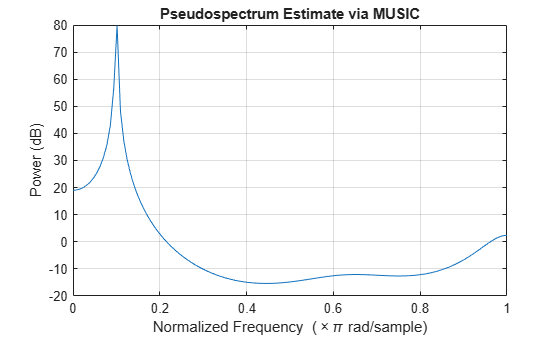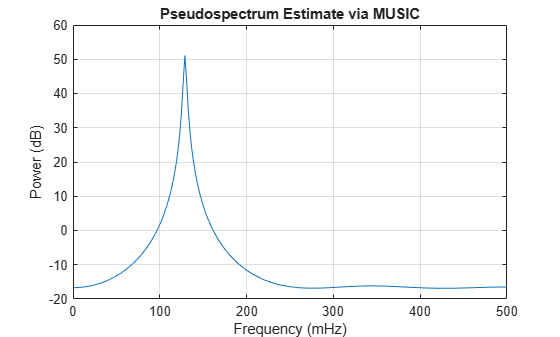Documentation

### This is machine translation

Mouseover text to see original. Click the button below to return to the English version of the page.

# pmusic

Pseudospectrum using MUSIC algorithm

## Syntax

```[S,w] = pmusic(x,p) [S,w] = pmusic(x,p,w) [S,w] = pmusic(...,nfft) [S,f] = pmusic(x,p,nfft,fs) [S,f] = pmusic(x,p,f,fs) [S,f] = pmusic(...,'corr') [S,f] = pmusic(x,p,nfft,fs,nwin,noverlap) [...] = pmusic(...,freqrange) [...,v,e] = pmusic(...) pmusic(...) ```

## Description

`[S,w] = pmusic(x,p)` implements the MUSIC (Multiple Signal Classification) algorithm and returns `S`, the pseudospectrum estimate of the input signal `x`, and a vector `w` of normalized frequencies (in rad/sample) at which the pseudospectrum is evaluated. The pseudospectrum is calculated using estimates of the eigenvectors of a correlation matrix associated with the input data `x`, where `x` is specified as either:

• A row or column vector representing one observation of the signal

• A rectangular array for which each row of `x` represents a separate observation of the signal (for example, each row is one output of an array of sensors, as in array processing), such that `x'*x` is an estimate of the correlation matrix

### Note

You can use the output of `corrmtx` to generate such an array `x`.

You can specify the second input argument `p` as either:

• A scalar integer. In this case, the signal subspace dimension is `p`.

• A two-element vector. In this case, `p(2)`, the second element of `p`, represents a threshold that is multiplied by λmin, the smallest estimated eigenvalue of the signal's correlation matrix. Eigenvalues below the threshold λmin`*p(2)` are assigned to the noise subspace. In this case, `p(1)` specifies the maximum dimension of the signal subspace.

### Note

If the inputs to `pmusic` are real sinusoids, set the value of `p` to double the number of input signals. If the inputs are complex sinusoids, set `p` equal to the number of inputs.

The extra threshold parameter in the second entry in `p` provides you more flexibility and control in assigning the noise and signal subspaces.

`S` and `w` have the same length. In general, the length of the FFT and the values of the input `x` determine the length of the computed `S` and the range of the corresponding normalized frequencies. The following table indicates the length of `S` (and `w`) and the range of the corresponding normalized frequencies for this syntax.

S Characteristics for an FFT Length of 256 (Default)

Real/Complex Input DataLength of S and wRange of the Corresponding Normalized Frequencies

Real-valued

129

[0, π]

Complex-valued

256

[0, 2π)

`[S,w] = pmusic(x,p,w)` returns the pseudospectrum in the vector `S` computed at the normalized frequencies specified in vector `w`. The vector `w` must have two or more elements, because otherwise the function interprets it as `nfft`

`[S,w] = pmusic(...,nfft)` specifies the integer length of the FFT `nfft` used to estimate the pseudospectrum. The default value for `nfft` (entered as an empty vector `[]`) is 256.

The following table indicates the length of `S` and `w`, and the frequency range for `w` in this syntax.

S and Frequency Vector Characteristics

Real/Complex Input Datanfft Even/OddLength of S and wRange of w

Real-valued

Even

`(nfft/2 + 1)`

[0, π]

Real-valued

Odd

`(nfft + 1)/2`

[0, π)

Complex-valued

Even or odd

`nfft`

[0, 2π)

`[S,f] = pmusic(x,p,nfft,fs)` returns the pseudospectrum in the vector `S` evaluated at the corresponding vector of frequencies `f` (in Hz). You supply the sampling frequency `fs` in Hz. If you specify `fs` with the empty vector `[]`, the sampling frequency defaults to 1 Hz.

The frequency range for `f` depends on `nfft`, `fs`, and the values of the input `x`. The length of `S` (and `f`) is the same as in the S and Frequency Vector Characteristics above. The following table indicates the frequency range for `f` for this syntax.

S and Frequency Vector Characteristics with fs Specified

Real/Complex Input Data

nfft Even/Odd

Range of f

Real-valued

Even

[0, `fs`/2]

Real-valued

Odd

[0, `fs`/2)

Complex-valued

Even or odd

[0, `fs`)

`[S,f] = pmusic(x,p,f,fs)` returns the pseudospectrum in the vector `S` computed at the frequencies specified in vector `f`. The vector `f` must have two or more elements, because otherwise the function interprets it as `nfft`

`[S,f] = pmusic(...,'corr')` forces the input argument `x` to be interpreted as a correlation matrix rather than matrix of signal data. For this syntax `x` must be a square matrix, and all of its eigenvalues must be nonnegative.

`[S,f] = pmusic(x,p,nfft,fs,nwin,noverlap)` allows you to specify `nwin`, a scalar integer indicating a rectangular window length, or a real-valued vector specifying window coefficients. Use the scalar integer `noverlap` in conjunction with `nwin` to specify the number of input sample points by which successive windows overlap. `noverlap` is not used if `x` is a matrix. The default value for `nwin` is 2 × `p(1)` and `noverlap` is `nwin` – 1.

With this syntax, the input data `x` is segmented and windowed before the matrix used to estimate the correlation matrix eigenvalues is formulated. The segmentation of the data depends on `nwin`, `noverlap`, and the form of `x`. Comments on the resulting windowed segments are described in the following table.

Windowed Data Depending on x and nwin

Input data x

Form of nwin

Windowed Data

Data vector

Scalar

Length is `nwin`

Data vector

Vector of coefficients

Length is `length(nwin)`

Data matrix

Scalar

Data is not windowed.

Data matrix

Vector of coefficients

`length(nwin)` must be the same as the column length of `x`, and `noverlap` is not used.

See the Eigenvector Length Depending on Input Data and Syntax below for related information on this syntax.

### Note

The arguments `nwin` and `noverlap` are ignored when you include `'corr'` in the syntax.

`[...] = pmusic(...,freqrange)` specifies the range of frequency values to include in `f` or `w`. `freqrange` can be either:

• `'half'` — returns half the spectrum for a real input signal, `x`. If `nfft` is even, then `S` has length `nfft`/2 + 1 and is computed over the interval [0,π]. If `nfft` is odd, the length of `S` is (`nfft` + 1)/2 and the frequency interval is [0,π). When your specify `fs`, the intervals are [0,`fs`/2) and [0,`fs`/2] for even and odd `nfft`, respectively.

• `'whole'` — returns the whole spectrum for either real or complex input, `x`. In this case, `S` has length `nfft` and is computed over the interval [0,2π). When you specify `fs`, the frequency interval is [0,`fs`).

• `'centered'` — returns the centered whole spectrum for either real or complex input, `x`. In this case, `S` has length `nfft` and is computed over the interval (–π,π] for even `nfft` and (–π,π) for odd `nfft`. When you specify `fs`, the frequency intervals are (–`fs`/2,`fs`/2] and (–`fs`/2,`fs`/2) for even and odd `nfft`, respectively.

### Note

You can put the arguments `freqrange` or `'corr'` anywhere in the input argument list after `p`.

`[...,v,e] = pmusic(...)` returns the matrix `v` of noise eigenvectors, along with the associated eigenvalues in the vector `e`. The columns of `v` span the noise subspace of dimension `size(v,2)`. The dimension of the signal subspace is `size(v,1)-size(v,2)`. For this syntax, `e` is a vector of estimated eigenvalues of the correlation matrix.

`pmusic(...)` with no output arguments plots the pseudospectrum in the current figure window.

## Examples

collapse all

This example analyzes a signal vector, `x`, assuming that two real sinusoidal components are present in the signal subspace. In this case, the dimension of the signal subspace is 4, because each real sinusoid is the sum of two complex exponentials.

```n = 0:199; x = cos(0.257*pi*n) + sin(0.2*pi*n) + 0.01*randn(size(n)); pmusic(x,4) % Set p to 4 because there are two real inputs```This example analyzes the same signal vector, `x`, with an eigenvalue cutoff of 10% above the minimum. Setting `p(1) = Inf` forces the signal/noise subspace decision to be based on the threshold parameter, `p(2)`. Specify the eigenvectors of length 7 using the `nwin` argument, and set the sampling frequency, `fs`, to 8 kHz:

```rng default n = 0:199; x = cos(0.257*pi*n) + sin(0.2*pi*n) + 0.01*randn(size(n)); [P,f] = pmusic(x,[Inf,1.1],[],8000,7); % Window length = 7 plot(f,20*log10(abs(P))) xlabel 'Frequency (Hz)', ylabel 'Power (dB)' title 'Pseudospectrum Estimate via MUSIC', grid on```Supply a positive definite correlation matrix, `R`, for estimating the spectral density. Use the default 256 samples.

```R = toeplitz(cos(0.1*pi*(0:6))) + 0.1*eye(7); pmusic(R,4,'corr')```Enter a signal data matrix, `Xm`, generated from data using `corrmtx`.

```n = 0:699; x = cos(0.257*pi*(n)) + 0.1*randn(size(n)); Xm = corrmtx(x,7,'modified'); pmusic(Xm,2)```Use the same signal, but let `pmusic` form the 100-by-7 data matrix using its windowing input arguments. In addition, specify an FFT of length 512.

```n = 0:699; x = cos(0.257*pi*(n)) + 0.1*randn(size(n)); [PP,ff] = pmusic(x,2,512,[],7,0); pmusic(x,2,512,[],7,0)```## Tips

In the process of estimating the pseudospectrum, `pmusic` computes the noise and signal subspaces from the estimated eigenvectors vj and eigenvalues λj of the signal's correlation matrix. The smallest of these eigenvalues is used in conjunction with the threshold parameter `p(2)` to affect the dimension of the noise subspace in some cases.

The length n of the eigenvectors computed by `pmusic` is the sum of the dimensions of the signal and noise subspaces. This eigenvector length depends on your input (signal data or correlation matrix) and the syntax you use.

The following table summarizes the dependency of the eigenvector length on the input argument.

Eigenvector Length Depending on Input Data and Syntax

Form of Input Data x

Length n of Eigenvectors

Row or column vector

`nwin` is specified as a scalar integer.

`nwin`

Row or column vector

`nwin` is specified as a vector.

`length(nwin)`

Row or column vector

`nwin` is not specified.

2 × `p(1)`

l-by-m matrix

If `nwin` is specified as a scalar, it is not used. If `nwin` is specified as a vector, `length(nwin)` must equal m.

m

m-by-m nonnegative definite matrix

`'corr'` is specified and `nwin` is not used.

m

You should specify `nwin` > `p(1)` or `length(nwin)` > `p(1)` if you want `p(2)` > `1` to have any effect.

## Algorithms

The name MUSIC is an acronym for MUltiple SIgnal Classification. The MUSIC algorithm estimates the pseudospectrum from a signal or a correlation matrix using Schmidt's eigenspace analysis method . The algorithm performs eigenspace analysis of the signal's correlation matrix in order to estimate the signal's frequency content. This algorithm is particularly suitable for signals that are the sum of sinusoids with additive white Gaussian noise. The eigenvalues and eigenvectors of the signal's correlation matrix are estimated if you don't supply the correlation matrix.

The MUSIC pseudospectrum estimate is given by

`${P}_{\text{MUSIC}}\left(f\right)=\frac{1}{{e}^{H}\left(f\right)\left(\sum _{k=p+1}^{N}{\text{v}}_{k}{\text{v}}_{k}^{H}\right)e\left(f\right)}=\frac{1}{\sum _{k=p+1}^{N}|{\text{v}}_{k}^{H}e\left(f\right){|}^{2}}$`

where N is the dimension of the eigenvectors and vk is the k-th eigenvector of the correlation matrix. The integer p is the dimension of the signal subspace, so the eigenvectors vk used in the sum correspond to the smallest eigenvalues and also span the noise subspace. The vector e(f) consists of complex exponentials, so the inner product

`${v}_{k}^{H}e\left(f\right)$`

amounts to a Fourier transform. This is used for computation of the pseudospectrum estimate. The FFT is computed for each vk and then the squared magnitudes are summed.

## References

 Marple, S. Lawrence. Digital Spectral Analysis. Englewood Cliffs, NJ: Prentice-Hall, 1987, pp. 373–378.

 Schmidt, R. O. “Multiple Emitter Location and Signal Parameter Estimation.” IEEE® Transactions on Antennas and Propagation. Vol. AP-34, March, 1986, pp. 276–280.

 Stoica, Petre, and Randolph L. Moses. Spectral Analysis of Signals. Upper Saddle River, NJ: Prentice Hall, 2005.